Home
Hostname: page-component-59b7f5684b-b2xwp Total loading time: 1.162 Render date: 2022-10-03T18:25:44.746Z Has data issue: true Feature Flags: { "shouldUseShareProductTool": true, "shouldUseHypothesis": true, "isUnsiloEnabled": true, "useRatesEcommerce": false, "displayNetworkTab": true, "displayNetworkMapGraph": false, "useSa": true } hasContentIssue true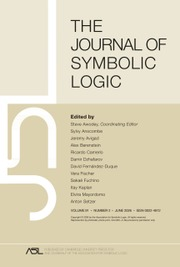The Journal of Symbolic Logic

# RELATIONSHIPS BETWEEN COMPUTABILITY-THEORETIC PROPERTIES OF PROBLEMS

Published online by Cambridge University Press:  05 October 2020

## Abstract

A problem is a multivalued function from a set of instances to a set of solutions. We consider only instances and solutions coded by sets of integers. A problem admits preservation of some computability-theoretic weakness property if every computable instance of the problem admits a solution relative to which the property holds. For example, cone avoidance is the ability, given a noncomputable set A and a computable instance of a problem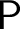${\mathsf {P}}$ , to find a solution relative to which A is still noncomputable.

In this article, we compare relativized versions of computability-theoretic notions of preservation which have been studied in reverse mathematics, and prove that the ones which were not already separated by natural statements in the literature actually coincide. In particular, we prove that it is equivalent to admit avoidance of one cone, of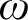$\omega$ cones, of one hyperimmunity or of one non-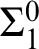$\Sigma ^{0}_1$ definition. We also prove that the hierarchies of preservation of hyperimmunity and non-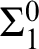$\Sigma ^{0}_1$ definitions coincide. On the other hand, none of these notions coincide in a nonrelativized setting.

## MSC classification

Type
Article
Information
The Journal of Symbolic Logic , March 2022 , pp. 47 - 71
© The Author(s), 2020. Published by Cambridge University Press on behalf of The Association for Symbolic Logic

## Access options

Get access to the full version of this content by using one of the access options below. (Log in options will check for institutional or personal access. Content may require purchase if you do not have access.)

## References

Andrews, U., et al, On cototality and the skip operator in the enumeration degrees. Transactions of the American Mathematical Society , vol. 372 (2019), no. 3, pp. 16311670.CrossRefGoogle Scholar
Arslanov, M. M., Kalimullin, I. S., and Kuper, S. B., Splitting properties of total enumeration degrees . Algebra Logika , vol. 42(2003), no. 1, pp. 325, 125.CrossRefGoogle Scholar
Cholak, P. A., et al, Free sets and reverse mathematics . Reverse Mathematics , vol. 21 (2001), pp. 104119.Google Scholar
Cholak, P. A., Jockusch, C. G., and Slaman, T. A., On the strength of Ramsey’s theorem for pairs , this Journal, vol. 66 (2001), no. 01, pp. 155.Google Scholar
Cholak, P. A. and Patey, L., Thin set theorems and cone avoidance. to appear, 2019.CrossRefGoogle Scholar
Dekker, J. C. E. and Myhill, J., Retraceable sets. Canadian Journal of Mathematics , vol. 10 (1958), pp. 357373.CrossRefGoogle Scholar
Downey, R., Nies, A., Weber, R., and Yu, L., Lowness and${\varPi}_2^0$ nullsets , this Journal, vol. 71 (2006), no. 3, pp. 10441052.Google Scholar
Dzhafarov, D. D. and Jockusch, C. G., Ramsey’s theorem and cone avoidance , this Journal, vol. 74 (2009), no. 2, pp. 557578.Google Scholar
Friedberg, R. M. and Rogers, H. Jr. Reducibility and completeness for sets of integers . Mathematical Logic Quarterly , vol5 (1959), pp. 117125.Google Scholar
Groszek, M. J and Slaman, T. A., Moduli of Computation (talk). Conference on Logic, Computability and Randomness, Buenos Aires, Argentina, 2007.Google Scholar
Hirschfeldt, D. R., et al, The strength of some combinatorial principles related to Ramsey’s theorem for pairs , Computational Prospects of Infinity, Part II: Presented Talks , Lecture Notes Series, Institute for Mathematical Sciences, National University of Singapore, 15, World Scientific Publishing, Hackensack, NJ, 2008, pp. 143161.CrossRefGoogle Scholar
Jockusch, C. G., Ramsey’s theorem and recursion theory , this Journal, vol. 37 (1972), no. 2, pp. 268280.Google Scholar
Jockusch, C. G. and Robert, I. S.,${\Pi}_0^1$ classes and degrees of theories . Transactions of the American Mathematical Society , vol. 173 (1972), pp. 3356.Google Scholar
Jockusch, C. G. Jr. Semirecursive sets and positive reducibility . Transactions of the American Mathematical Society , vol. 131 (1968), pp. 420436.CrossRefGoogle Scholar
Jockusch, C. G. Jr. Reducibilities In Recursive Function Theory , (Ph.D thesis), Massachusetts Institute of Technology, ProQuest LLC, Ann Arbor, MI, 1966.Google Scholar
Kautz, S. M., Degrees of random sets , Ph.D thesis, Citeseer, 1991.Google Scholar
Kihara, T., Ng, K. M., and Pauly, A., Enumeration degrees and non-metrizable topology, 2019, arXiv:1904.04107 [math.GN].Google Scholar
Lerman, M., Solomon, R., and Towsner, H., Separating principles below Ramsey’s theorem for pairs . Journal of Mathematical Logic , vol. 13 (2013), no. 02, p. 1350007.Google Scholar
Liu, L,${RT}_2^2$ does not imply WKL 0 , this Journal, vol. 77 (2012), no. 2, pp. 609620.Google Scholar
Liu, L, Cone avoiding closed sets . Transactions of the American Mathematical Society , vol. 367 (2015), no. 3, pp. 16091630.CrossRefGoogle Scholar
Miller, J. S. and Soskova, M. I., Density of the cototal enumeration degrees . Annals of Pure and Applied Logic , vol. 169 (2018), no. 5, pp. 450462.CrossRefGoogle Scholar
Patey, L., Combinatorial weaknesses of Ramseyan principles, In preparation. 2015. Available at http://ludovicpatey.com/media/research/combinatorial-weaknesses-draft.pdf.Google Scholar
Patey, L., Partial orders and immunity in reverse mathematics. Conference on Computability in Europe, 2016, Springer, Cham.CrossRefGoogle Scholar
Patey, L., The weakness of being cohesive, thin or free in reverse mathematics . Israel Journal of Mathematics , vol. 216 (2016), no. 2, pp. 905955.CrossRefGoogle Scholar
Patey, L., Iterative forcing and hyperimmunity in reverse mathematics . Computability , vol. 6 (2017), no. 3, pp. 209221.CrossRefGoogle Scholar
Patey, L., Ramsey-like theorems and moduli of computation. arXiv preprint, 2019, arXiv:1901.04388.CrossRefGoogle Scholar
Rice, B.. Thin set for pairs implies DNR. Notre Dame J. Formal Logic, to appear, 2015.Google Scholar
Rosenstein, J. G., Linear orderings volume 98 of Pure and Applied Mathematics. Academic Press, Inc. [Harcourt Brace Jovanovich, Publishers], New York, NY-London, UK, 1982.Google Scholar
Seetapun, D. and Slaman, T. A., On the strength of Ramsey’s theorem . Notre Dame Journal of Formal Logic , vol. 36 (1995), no. 4, pp. 570582.Google Scholar
Selman, A. L., Arithmetical reducibilities . Zeitschrift für Mathematische Logik und Grundlagen der Mathematik , vol. 17 (1971), pp. 335350.CrossRefGoogle Scholar
Simpson, S. G., Subsystems of Second Order Arithmetic . Cambridge University Press, Cambridge, MA, 2009.CrossRefGoogle Scholar
Terwijn, S. A. and Zambella, D., Computational randomness and lowness , this Journal, vol. 66 (2001), no. 3, pp. 11991205.Google Scholar
Wang, W., The definability strength of combinatorial principles, to appear, 2014. Available at http://arxiv.org/abs/1408.1465.Google Scholar
Wang, W., Some logically weak Ramseyan theorems . Advances in Mathematics , vol. 261 (2014), pp. 125.CrossRefGoogle Scholar
2
Cited by

# Save article to Kindle

To save this article to your Kindle, first ensure coreplatform@cambridge.org is added to your Approved Personal Document E-mail List under your Personal Document Settings on the Manage Your Content and Devices page of your Amazon account. Then enter the ‘name’ part of your Kindle email address below. Find out more about saving to your Kindle.

Note you can select to save to either the @free.kindle.com or @kindle.com variations. ‘@free.kindle.com’ emails are free but can only be saved to your device when it is connected to wi-fi. ‘@kindle.com’ emails can be delivered even when you are not connected to wi-fi, but note that service fees apply.

Find out more about the Kindle Personal Document Service.

RELATIONSHIPS BETWEEN COMPUTABILITY-THEORETIC PROPERTIES OF PROBLEMS
Available formats
×

# Save article to Dropbox

To save this article to your Dropbox account, please select one or more formats and confirm that you agree to abide by our usage policies. If this is the first time you used this feature, you will be asked to authorise Cambridge Core to connect with your Dropbox account. Find out more about saving content to Dropbox.

RELATIONSHIPS BETWEEN COMPUTABILITY-THEORETIC PROPERTIES OF PROBLEMS
Available formats
×

# Save article to Google Drive

To save this article to your Google Drive account, please select one or more formats and confirm that you agree to abide by our usage policies. If this is the first time you used this feature, you will be asked to authorise Cambridge Core to connect with your Google Drive account. Find out more about saving content to Google Drive.

RELATIONSHIPS BETWEEN COMPUTABILITY-THEORETIC PROPERTIES OF PROBLEMS
Available formats
×
×